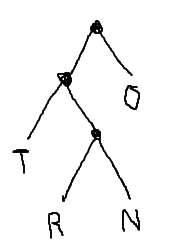# Quiz 1 Solutions

[4 points for showing up]

1. Using 7-bit signed (two’s complement) binary numbers, what is the largest positive number? What is the smallest negative number? [6 points]

In 7-bit two’s complement, the column values are:

``````  ___  ___  ___  ___  ___  ___  ___
-64  32   16    8    4    2    1``````

So the largest positive number is 0111111 = 63 and the most negative number is 1000000 = -64.

2. Convert the following 16-bit binary number into hexadecimal. [6 points]

``````8 4 2 1  8 4 2 1  8 4 2 1  8 4 2 1
-------  -------  -------  -------
0 1 1 1  1 1 1 1  0 0 1 1  1 0 1 0

7       F       3       A``````
3. Add and verify the following unsigned binary numbers. [6 points]

``````  1 1 1 1 1                      1     1
1 0 1 1 1 1  = 47                1 1 0 1 1 1  = 55
+ 0 1 1 1 0 1  = 29              + 1 0 0 1 0 0  = 36
--------------   --              --------------   --
1 0 0 1 1 0 0  = 76              1 0 1 1 0 1 1  = 91

32  8   2                        32  8   2
64  16  4   1                    64  16  4   1``````
4. Suppose we need to send a text message uses just 15 distinct characters. How many bits per character are required if we’re using a fixed encoding? [6 points]

We need 4 bits per character, which allows 16 distinct characters to be represented.

5. Draw a binary tree that corresponds to the following variable-width encoding of four characters. The characters should appear in boxes at the leaves. Branch left on a zero, or right on a one. [6 points]

``````T 00
R 010
N 011
O 1   ``````6. Use the character encoding from the previous question to decode the following word: [6 points]

``````0 0,1,0 1 0,1,0 1 1,0 0,1
T O     R O     N   T O``````